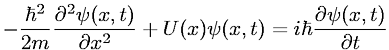Equations > Physics > Modern Physics > Time-dependent, one-dimensional Schr

Time-dependent, one-dimensional SchrLatex Code:

MathML Code:

 $-\frac\hslash {}^{2}2m\frac{\partial }^{2}\psi \left(x,t\right)\partial {x}^{2}+U\left(x\right)\psi \left(x,t\right)=i\hslash \frac\partial \psi \left(x,t\right)\partial t$

MathType 5.0: